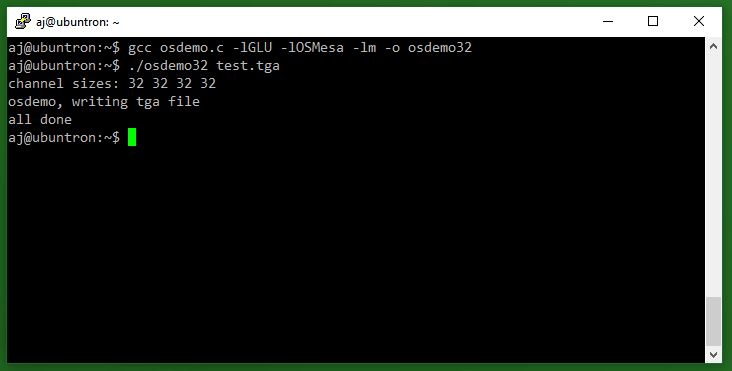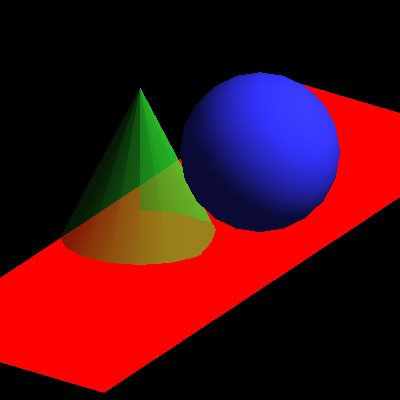Ever tried to render something with openGL on a linux server that has no x11 installed nor has a physical gpu connected? I searched for a long time for a solution that would work almost out of the box and the first solution i could find was actually installing x11, mesa3d and a dummy x-display. Via putty that has x11-forwarding enabled it is possible to run x-applications just fine and even render with openGL thanks to mesa in software mode!

But as soon as you close the ssh connection x11 stops and after that x-applications and openGL won’t be able to connect to a display! So after every server restart openGL won’t work anymore…

So why do you need a display or x11 if you want to render offscreen?

The answer is you don’t! Here is a quick test:

## 1. Install libraries

I set up a virtual machine in virtual box based on ubuntu server 17.04 and installed the following libs to get started:

• mesa for openGL software rendering
• osmesa for offscreen rendering
• glu1-mesa for some glu 🙂
• gcc to compile c
```sudo apt install mesa-common-dev sudo apt install libosmesa6-dev sudo apt install libglu1-mesa-dev sudo apt install gcc```

## 2. Create source file

Now lets create the osmesa demo which i have modified slightly to compile out of the box:

`sudo nano osdemo.c`

Paste the following code in nano:

```/* based on:   https://github.com/JoakimSoderberg/mesademos/blob/master/src/osdemos/osdemo32.c */   #include <stdio.h> #include <stdlib.h> #include <GL/osmesa.h> #include <GL/glu.h> //replaced by default header in linux   #define SAVE_TARGA   #define WIDTH 400 #define HEIGHT 400   static void render_image( void ) { GLfloat light_ambient[] = { 0.0, 0.0, 0.0, 1.0 }; GLfloat light_diffuse[] = { 1.0, 1.0, 1.0, 1.0 }; GLfloat light_specular[] = { 1.0, 1.0, 1.0, 1.0 }; GLfloat light_position[] = { 1.0, 1.0, 1.0, 0.0 }; GLfloat red_mat[] = { 1.0, 0.2, 0.2, 1.0 }; GLfloat green_mat[] = { 0.2, 1.0, 0.2, 0.5 }; GLfloat blue_mat[] = { 0.2, 0.2, 1.0, 1.0 }; GLfloat white_mat[] = { 1.0, 1.0, 1.0, 1.0 }; GLfloat purple_mat[] = { 1.0, 0.2, 1.0, 1.0 }; GLUquadricObj *qobj = gluNewQuadric();   glLightfv(GL_LIGHT0, GL_AMBIENT, light_ambient); glLightfv(GL_LIGHT0, GL_DIFFUSE, light_diffuse); glLightfv(GL_LIGHT0, GL_SPECULAR, light_specular); glLightfv(GL_LIGHT0, GL_POSITION, light_position);   glEnable(GL_LIGHTING); glEnable(GL_LIGHT0); glEnable(GL_DEPTH_TEST);   glMatrixMode(GL_PROJECTION); glLoadIdentity(); glOrtho(-2.5, 2.5, -2.5, 2.5, -10.0, 10.0); glMatrixMode(GL_MODELVIEW);   glClear( GL_COLOR_BUFFER_BIT | GL_DEPTH_BUFFER_BIT );   glPushMatrix(); glRotatef(20.0, 1.0, 0.0, 0.0);   #if 0 glPushMatrix(); glTranslatef(-0.75, 0.5, 0.0); glRotatef(90.0, 1.0, 0.0, 0.0); glMaterialfv( GL_FRONT_AND_BACK, GL_AMBIENT_AND_DIFFUSE, red_mat ); glutSolidTorus(0.275, 0.85, 20, 20); glPopMatrix(); #endif   /* red square */ glPushMatrix(); glTranslatef(0.0, -0.5, 0.0); glRotatef(90, 1, 0.5, 0); glScalef(3, 3, 3); glDisable(GL_LIGHTING); glColor4f(1, 0, 0, 0.5); glBegin(GL_POLYGON); glVertex2f(-1, -1); glVertex2f( 1, -1); glVertex2f( 1, 1); glVertex2f(-1, 1); glEnd(); glEnable(GL_LIGHTING); glPopMatrix();   #if 0 /* green square */ glPushMatrix(); glTranslatef(0.0, 0.5, 0.1); glDisable(GL_LIGHTING); glColor3f(0, 1, 0); glBegin(GL_POLYGON); glVertex2f(-1, -1); glVertex2f( 1, -1); glVertex2f( 1, 1); glVertex2f(-1, 1); glEnd(); glEnable(GL_LIGHTING); glPopMatrix(); /* blue square */ glPushMatrix(); glTranslatef(0.75, 0.5, 0.3); glDisable(GL_LIGHTING); glColor3f(0, 0, 0.5); glBegin(GL_POLYGON); glVertex2f(-1, -1); glVertex2f( 1, -1); glVertex2f( 1, 1); glVertex2f(-1, 1); glEnd(); glEnable(GL_LIGHTING); glPopMatrix(); #endif glPushMatrix(); glTranslatef(-0.75, -0.5, 0.0); glRotatef(270.0, 1.0, 0.0, 0.0); glMaterialfv( GL_FRONT_AND_BACK, GL_AMBIENT_AND_DIFFUSE, green_mat ); glColor4f(0,1,0,0.5); glEnable(GL_BLEND); glBlendFunc(GL_SRC_ALPHA, GL_ONE_MINUS_SRC_ALPHA); gluCylinder(qobj, 1.0, 0.0, 2.0, 16, 1); glDisable(GL_BLEND); glPopMatrix();   glPushMatrix(); glTranslatef(0.75, 1.0, 1.0); glMaterialfv( GL_FRONT_AND_BACK, GL_AMBIENT_AND_DIFFUSE, blue_mat ); gluSphere(qobj, 1.0, 20, 20); glPopMatrix();   glPopMatrix();   /* This is very important!!! * Make sure buffered commands are finished!!! */ glFinish();   gluDeleteQuadric(qobj);   { GLint r, g, b, a; glGetIntegerv(GL_RED_BITS, &r); glGetIntegerv(GL_GREEN_BITS, &g); glGetIntegerv(GL_BLUE_BITS, &b); glGetIntegerv(GL_ALPHA_BITS, &a); printf("channel sizes: %d %d %d %d\n", r, g, b, a); } }       static void write_targa(const char *filename, const GLfloat *buffer, int width, int height) { FILE *f = fopen( filename, "w" ); if (f) { int i, x, y; const GLfloat *ptr = buffer; printf ("osdemo, writing tga file \n"); fputc (0x00, f); /* ID Length, 0 => No ID */ fputc (0x00, f); /* Color Map Type, 0 => No color map included */ fputc (0x02, f); /* Image Type, 2 => Uncompressed, True-color Image */ fputc (0x00, f); /* Next five bytes are about the color map entries */ fputc (0x00, f); /* 2 bytes Index, 2 bytes length, 1 byte size */ fputc (0x00, f); fputc (0x00, f); fputc (0x00, f); fputc (0x00, f); /* X-origin of Image */ fputc (0x00, f); fputc (0x00, f); /* Y-origin of Image */ fputc (0x00, f); fputc (WIDTH & 0xff, f); /* Image Width */ fputc ((WIDTH>>8) & 0xff, f); fputc (HEIGHT & 0xff, f); /* Image Height */ fputc ((HEIGHT>>8) & 0xff, f); fputc (0x18, f); /* Pixel Depth, 0x18 => 24 Bits */ fputc (0x20, f); /* Image Descriptor */ fclose(f); f = fopen( filename, "ab" ); /* reopen in binary append mode */ for (y=height-1; y>=0; y--) { for (x=0; x<width; x++) { int r, g, b; i = (y*width + x) * 4; r = (int) (ptr[i+0] * 255.0); g = (int) (ptr[i+1] * 255.0); b = (int) (ptr[i+2] * 255.0); if (r > 255) r = 255; if (g > 255) g = 255; if (b > 255) b = 255; fputc(b, f); /* write blue */ fputc(g, f); /* write green */ fputc(r, f); /* write red */ } } } }     static void write_ppm(const char *filename, const GLfloat *buffer, int width, int height) { const int binary = 0; FILE *f = fopen( filename, "w" ); if (f) { int i, x, y; const GLfloat *ptr = buffer; if (binary) { fprintf(f,"P6\n"); fprintf(f,"# ppm-file created by osdemo.c\n"); fprintf(f,"%i %i\n", width,height); fprintf(f,"255\n"); fclose(f); f = fopen( filename, "ab" ); /* reopen in binary append mode */ for (y=height-1; y>=0; y--) { for (x=0; x<width; x++) { int r, g, b; i = (y*width + x) * 4; r = (int) (ptr[i+0] * 255.0); g = (int) (ptr[i+1] * 255.0); b = (int) (ptr[i+2] * 255.0); if (r > 255) r = 255; if (g > 255) g = 255; if (b > 255) b = 255; fputc(r, f); /* write red */ fputc(g, f); /* write green */ fputc(b, f); /* write blue */ } } } else { /*ASCII*/ int counter = 0; fprintf(f,"P3\n"); fprintf(f,"# ascii ppm file created by osdemo.c\n"); fprintf(f,"%i %i\n", width, height); fprintf(f,"255\n"); for (y=height-1; y>=0; y--) { for (x=0; x<width; x++) { int r, g, b; i = (y*width + x) * 4; r = (int) (ptr[i+0] * 255.0); g = (int) (ptr[i+1] * 255.0); b = (int) (ptr[i+2] * 255.0); if (r > 255) r = 255; if (g > 255) g = 255; if (b > 255) b = 255; fprintf(f, " %3d %3d %3d", r, g, b); counter++; if (counter % 5 == 0) fprintf(f, "\n"); } } } fclose(f); } }     int main( int argc, char *argv[] ) { GLfloat *buffer;   /* Create an RGBA-mode context */ #if OSMESA_MAJOR_VERSION * 100 + OSMESA_MINOR_VERSION >= 305 /* specify Z, stencil, accum sizes */ OSMesaContext ctx = OSMesaCreateContextExt( GL_RGBA, 16, 0, 0, NULL ); #else OSMesaContext ctx = OSMesaCreateContext( GL_RGBA, NULL ); #endif if (!ctx) { printf("OSMesaCreateContext failed!\n"); return 0; }   /* Allocate the image buffer */ buffer = (GLfloat *) malloc( WIDTH * HEIGHT * 4 * sizeof(GLfloat)); if (!buffer) { printf("Alloc image buffer failed!\n"); return 0; }   /* Bind the buffer to the context and make it current */ if (!OSMesaMakeCurrent( ctx, buffer, GL_FLOAT, WIDTH, HEIGHT )) { printf("OSMesaMakeCurrent failed!\n"); return 0; }   render_image();   if (argc>1) { #ifdef SAVE_TARGA write_targa(argv, buffer, WIDTH, HEIGHT); #else write_ppm(argv, buffer, WIDTH, HEIGHT); #endif } else { printf("Specify a filename if you want to make an image file\n"); }   printf("all done\n");   /* free the image buffer */ free( buffer );   /* destroy the context */ OSMesaDestroyContext( ctx );   return 0; }```

The code is actually pretty straight forward. Just have a look at the main function. Create an OSMesaContext, allocate a buffer and call OSMesaMakeCurrent with the new buffer. After that you can call openGL functions and have fun. If you are done destroy the context and free the allocated buffer.
Now hit CTRL+O to save it and leave nano with CTRL+X.

## 3. Build executable and run the test

To create an executable program run the following command:

`gcc osdemo.c -lGLU -lOSMesa -lm -o osdemo32`

And try it out:

`./osdemo32 test.tga`

If everything went right you will see something like this:and the output image looks like this:That’s it! Rendering openGL stuff without x11, a physical display and gpu!